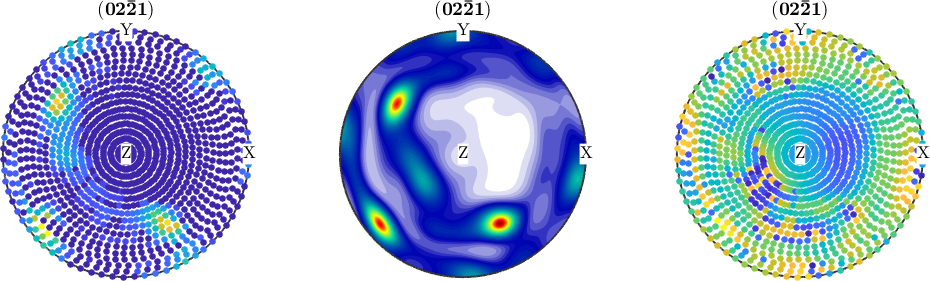Combined Plots edit page

Explains how to combine several plots, e.g. plotting on the top of an inverse pole figure some important crystal directions.

## General Principle

In order to tell MATLAB to plot one plot right on the top of an older plot one has to use the commands hold all and hold off. Let's demonstrate this using a simple example.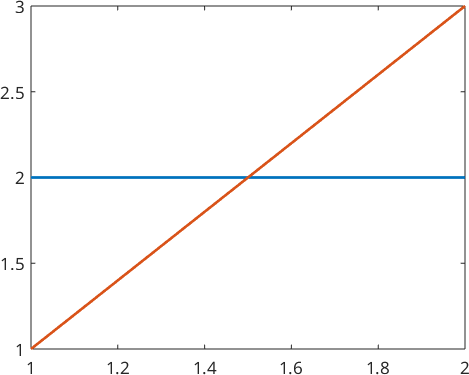## Combine Different EBSD Data

First, we want to show up two different EBSD data sets in one plot

let's simulate some EBSD data

plot them as a scatter plot in axis / angle parametrized orientation space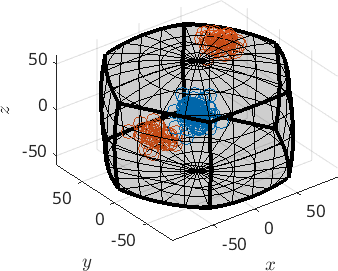a second way would be to superpose the pole figures of both EBSD data sets.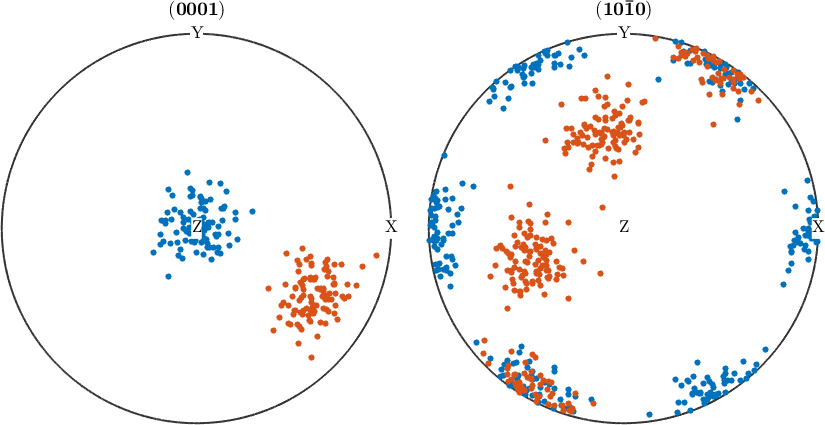## Combine countoured pole figures (smooth ODF plots) with EBSD Data Scatter Plots

You can also combine a contour plot of a model ODF with a scatter plot of single orientations.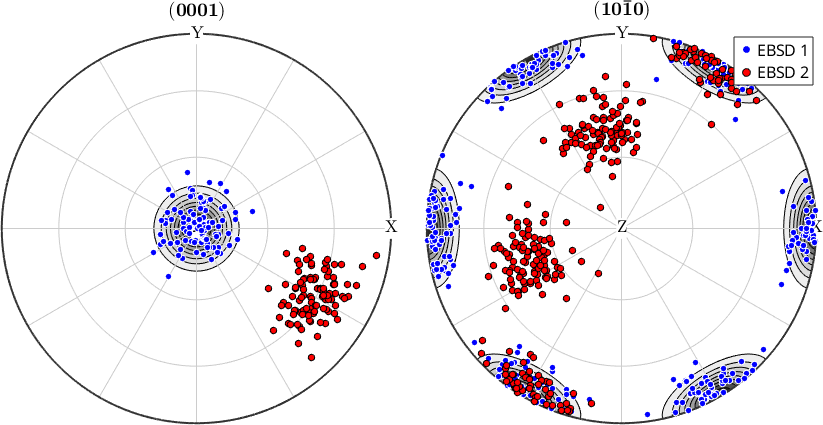and, of course, you can do the same with ODF plots: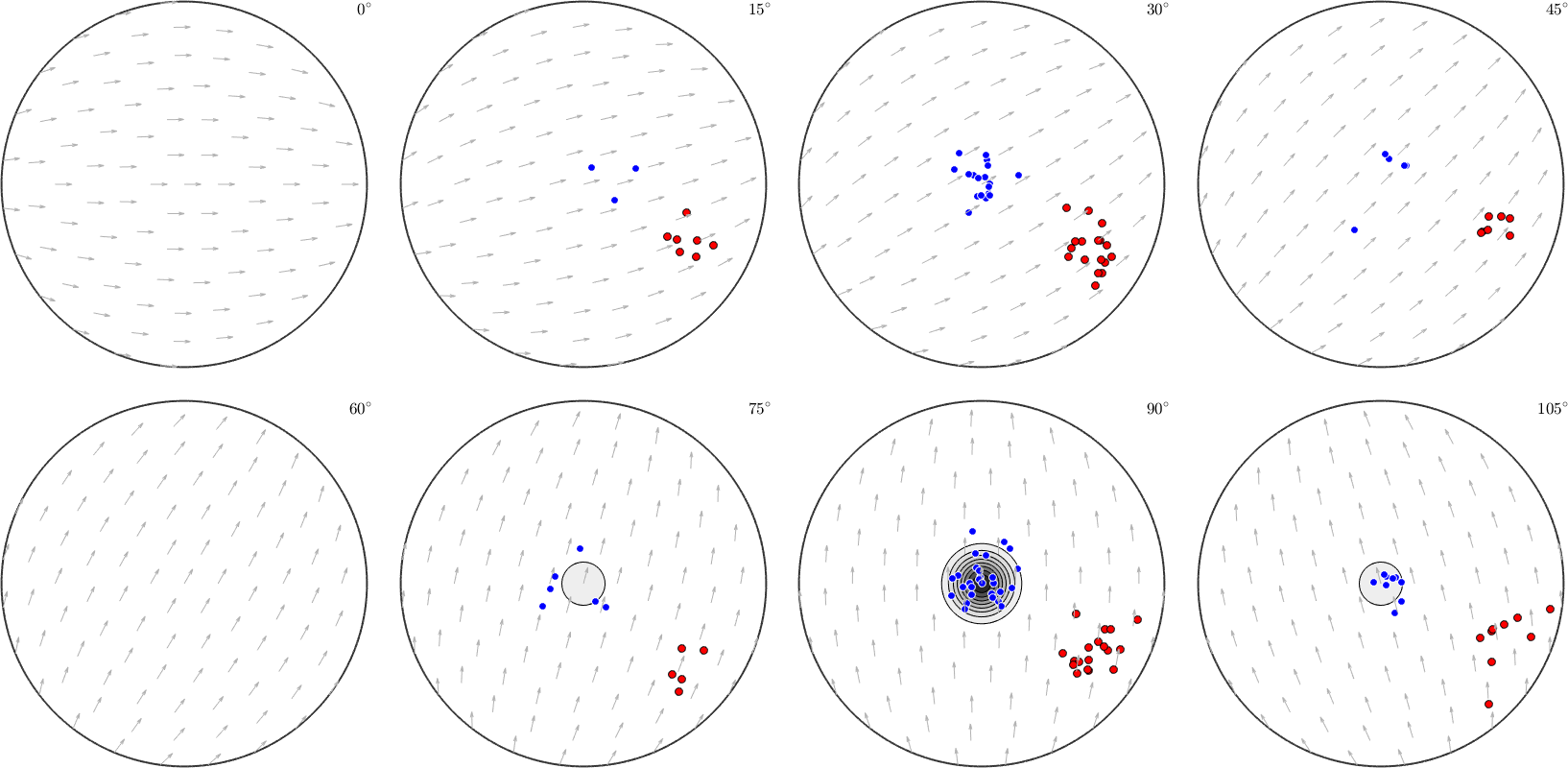## Add Miller Indices to an Inverse Pole Figure Plot

Next, we are going to add some Miller indices to an inverse pole figure plot.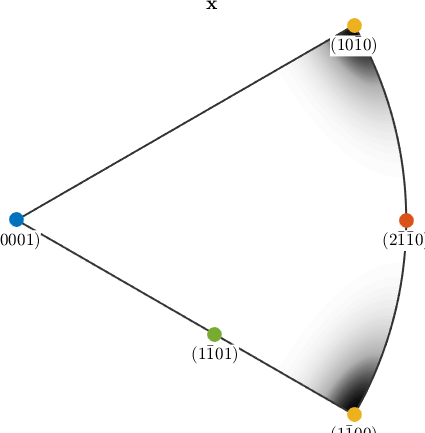## Combining different plots in one figure

The next example demonstrates how to arrange arbitrary plots into one figure

next, we compute an ODF out of them

now we want to plot the original data alongsite with the recalculated pole figures and with a difference plot